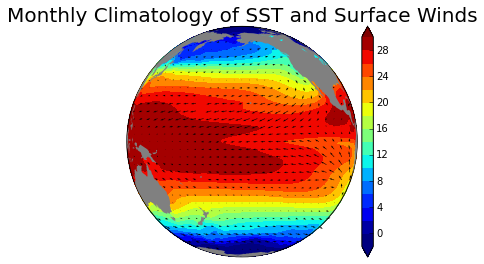# Fisseha Berhane, PhD

#### Data Scientist443-970-2353[email protected] CV Resume### Working with NetCDF data in Python

NetCDF (Network Common Data Form) data is a self-describing, portable, scalable and appendable machine-independent data format. More information can be found from Wikipedia. This data format is commonly used in Atmospheric science and Oceanography as it is convinient to store various variables of many dimensions. Here, let's see how to open netcdf data in python and generate monthly climatology of global sea surface temperature (SST) and near surface winds. First, we will import the required libraries, then we will open the monthly average global SST (which can be downloaded from here) and winds (which can be downloaded from here).

In :
% matplotlib inline

In :
from pylab import *
from optparse import OptionParser
import numpy as np

import matplotlib.pyplot as plt

from netCDF4 import Dataset  as netcdf # netcdf4-python module

In :
sst=netcdf(r'C:\Fish\Python\scripts\sst.mnmean.nc')

uwnd=netcdf( r'C:\Fish\Python\scripts\uwnd.mon.mean.nc')

vwnd=netcdf( r'C:\Fish\Python\scripts\vwnd.mon.mean.nc')

lons=sst['lon'][:]

lonu=uwnd['lon'][:]
lonv=vwnd['lon'][:]  #uwnd=vwnd

In :
# reverse temperature, u, v and latitude so they go from south to north.

latuv= vwnd['lat'][::-1]

lats=sst['lat'][::-1]

sst=sst['sst'][0:384,::-1,:]  #  to make it divisible by 12

u=uwnd['uwnd'][0:384,::-1,:]  #  to make it divisible by 12

v=vwnd['vwnd'][0:384,::-1,:]  #  to make it divisible by 12

sst , lons = addcyclic(sst, lons)

# shift data so lons go from -180 to 180 instead of 0 to 360.

sst,lons = shiftgrid(180.,sst,lons,start=False)

lons, lats = np.meshgrid(lons, lats)

In :
fig = plt.figure()
fig.patch.set_facecolor('white')

m = Basemap(resolution='c',projection='ortho',lat_0=0.,lon_0=-160.)

x, y = m(lons, lats)

# set desired contour levels.
clevs = np.arange(-30,30,5)

cs2 = m.contourf(x,y,sst.mean(axis=0),20,cmap=plt.cm.jet,vmin=0, vmax=30,extend='both')

cb = m.colorbar(cs2,"right", size="5%", pad='2%') #size='5%'

plt.title('Monthly Climatology of SST and Surface Winds',fontsize=20)

# plot wind vectors on projection grid.
# first, shift grid so it goes from -180 to 180 (instead of 0 to 360
# in longitude).  Otherwise, interpolation is messed up.

ugrid,newlons = shiftgrid(180.,u,lonu,start=False)
vgrid,newlons = shiftgrid(180.,v,lonv,start=False)

ugrid=ugrid.mean(axis=0)
vgrid=vgrid.mean(axis=0)

## transform vectors to projection grid. Make sure time is one unit

uproj,vproj,xx,yy = \

# now plot.

Q = m.quiver(xx,yy,uproj,vproj,scale=300,units='width')

qk = plt.quiverkey(Q, 0.5, 0.03, 5, r'$5 \frac{m}{s}$',
labelpos='E',
coordinates='figure',
fontproperties={'weight': 'light'})  #light

m.fillcontinents(color='gray',lake_color='aqua')

Out:We can also make animations using Matplotlib Animation as shown in this video.